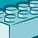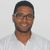# Search the Community

cancel
Showing results for
Did you mean:Contributor

## Fiscal year script

Dear,

I am running the script as provided in the exercise but it generates an error , i did not change it nor do I see a mistake in the script can. What am I missing?

error:

Orders << Orders Lines fetched: 13,142

The following error occurred:
Unexpected token: ')', expected one of: 'OPERATOR_PLUS', 'OPERATOR_MINUS', 'OPERATOR_MULTIPLICATION', 'OPERATOR_DIVISION', 'OPERATOR_STRING_CONCAT', 'like', 'and', ...

The error occurred here:
LET vStartDate = Num(YearStart(, -1)>>>>>>)<<<<<<

script from exercise  provided by qlik

Orders:
OrderRecordCounter,
OrderID,
Date(OrderDate) AS OrderDate,
CustomerID,
EmployeeID,
ShipperID,
FreightWeight,
OrderStatus,
LastUpdated
FROM [lib://DataFiles/Orders.qvd]
(qvd);

//vFiscalYearStartMonth - Tells the starting month of the Fiscal Year
//vStartDate - Starting date of the Calendar generation
//vEndDate - Ending date of the Calendar generation

LET vToday = Num('20-Mar-2015');

SET vFiscalYearStartMonth = 7;
LET vStartDate = Num(YearStart(\$(vToday), -1));
LET vEndDate = Num(YearEnd(\$(vToday)));

FiscalCalendar:
*,
Dual('Q' & Ceil(FiscalMonth/3), Ceil(FiscalMonth/3)) AS FiscalQuarter, // Fiscal Calendar Quarter
Dual(Text(Date(MonthEnd(OrderDate), 'MMM')), FiscalMonth) AS FiscalMonthName; // Fiscal Calendar Month Name

*,
Year(OrderDate)
as Year, // Standard Calendar Year
Month(OrderDate)
as Month, // Standard Calendar Month
Date(MonthEnd(OrderDate), 'MMM')
as MonthName, // Standard Calendar Month Name
Dual('Q' & Ceil(Month(OrderDate)/3), Ceil(Month(OrderDate)/3))
as Quarter, // Standard Calendar Quarter
Mod(Month(OrderDate) - \$(vFiscalYearStartMonth), 12) + 1
as FiscalMonth, // Fiscal Calendar Month
YearName(OrderDate, 0, \$(vFiscalYearStartMonth))
as FiscalYear; // Fiscal Calendar Year

Date(\$(vStartDate) + RangeSum(Peek('RowNum'), 1) - 1, 'DD-MMM-YYYY')
as OrderDate,
RangeSum(Peek('RowNum'), 1)
as RowNum
AutoGenerate vEndDate - vStartDate + 1;

Labels (1)
• ### Script of CreateFiscalCalendar in text file provided with excercise generates error

1 Solution

Accepted SolutionsMVP

@MirkO1  if I understood correctly

can you check your date format in LET vToday = Num('20-Mar-2015');

for example if your date default format is :

SET DateFormat='DD/MM/YYYY';

you can use :

LET vToday = floor(Num('20/04/2015'));

SET vFiscalYearStartMonth = 7;
LET vStartDate = floor(Num(YearStart(\$(vToday), -1)));
LET vEndDate = floor(Num(YearEnd(\$(vToday))));

debeug output:Regards,
Taoufiq ZARRA

"Please LIKE posts and "Accept as Solution" if the provided solution is helpful "

(you can mark up to 3 "solutions") 😉
2 RepliesMVP

@MirkO1  if I understood correctly

can you check your date format in LET vToday = Num('20-Mar-2015');

for example if your date default format is :

SET DateFormat='DD/MM/YYYY';

you can use :

LET vToday = floor(Num('20/04/2015'));

SET vFiscalYearStartMonth = 7;
LET vStartDate = floor(Num(YearStart(\$(vToday), -1)));
LET vEndDate = floor(Num(YearEnd(\$(vToday))));

debeug output:Regards,
Taoufiq ZARRA

"Please LIKE posts and "Accept as Solution" if the provided solution is helpful "

(you can mark up to 3 "solutions") 😉Contributor
Author

Dear Mr Taoufiq,

Many thanks this indeed works. Oddly this script was the orginal script from qliksense itself.

Best regards,

Mirko